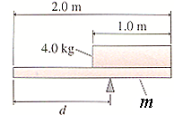# Problem: The two objects in the figure below are balanced on the pivot, with m = 1.7 kg. What is distance d?

###### FREE Expert Solution

A Moment (torque) is the product of Force and Distance.

$\overline{)\begin{array}{rcl}{\mathbf{F}}& {\mathbf{=}}& \mathbf{m}\mathbf{g}\end{array}}$

Let clockwise torque be positive and counter-clockwise torque be negative.

The system is in equilibrium. Therefore, the sum torque should be zero

$\overline{)\begin{array}{rcl}{\mathbf{\Sigma \tau }}& {\mathbf{=}}& {\mathbf{0}}\\ {\mathbf{\Sigma \tau }}_{\mathbf{cw}}\mathbf{-}{\mathbf{\Sigma \tau }}_{\mathbf{ccw}}& {\mathbf{=}}& {\mathbf{0}}\end{array}}$

84% (416 ratings)###### Problem Details

The two objects in the figure below are balanced on the pivot, with m = 1.7 kg. What is distance d?